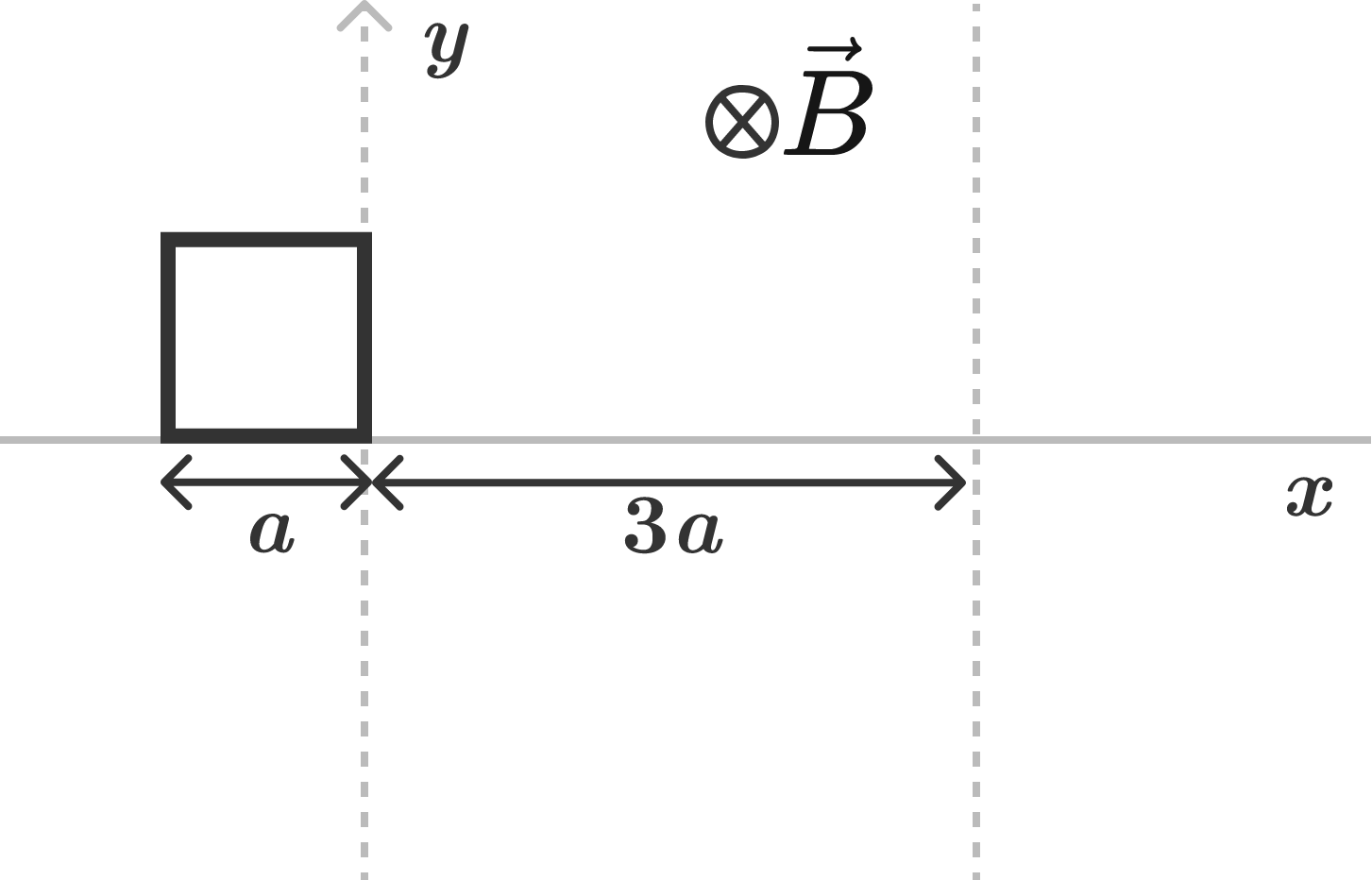# Magnetic fieldA square wire loop having side length $a$, mass $m$, and resistance $R$ is moving along the positive $x$-axis at a speed of $v_0$. It enters a uniform, steady magnetic field $\vec B = B_0 \big(-\widehat k \big)$ at $t = 0$ seconds, as shown in the figure.

Find the total amount of heat loss in the resistance.

Details and Assumptions:

• The magnitude of $v_0$ is sufficient that the loop comes out of the region of magnetic field with some speed.

• Neglect any type of energy loss other than the heat loss in resistance of the wires of the square.

• Take $v_0 = \dfrac{3B^2 a^3}{mR}$.

×